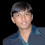.net & SQL Samples, programming tips and tricks, performance tips, guidelines, and best practices

## Monday, 11 March 2013

### SQL Server – Generating PERMUTATIONS using T-Sql

Were you ever asked to generate string Permutations using TSql? I was recently asked to do so, and the logic which I could manage to come up at that point is shared in the below script.

`DECLARE @Value AS VARCHAR(20) = 'ABCC' --Mention the text which is to be permuted`
`DECLARE @NoOfChars AS INT = LEN(@Value)`
`DECLARE @Permutations TABLE(Value VARCHAR(20)) --Make sure the size of this Value is equal to your input  string length (@Value)`
` `
`;WITH NumTally AS (--Prepare the Tally Table to separate each character of the Value.`
`  SELECT 1 Num`
`  UNION ALL`
`  SELECT `
`    Num + 1 `
`  FROM `
`    NumTally `
`  WHERE `
`    Num < @NoOfChars`
`),Chars AS ( --Separate the Characters`
`SELECT`
`  Num,`
`  SUBSTRING(@Value,Num,1) Chr`
`FROM`
`  NumTally  `
`)`
` `
`--Persist the Separated characters.`
`INSERT INTO @Permutations`
`SELECT Chr FROM Chars`
` `
`--Prepare Permutations`
`DECLARE @i AS INT = 1`
`WHILE(@i < @NoOfChars)`
`BEGIN`
` `
`  --Store the Permutations`
`  INSERT INTO @Permutations`
`  SELECT DISTINCT --Add DISTINCT if required else duplicate Permutations will be generated for Repeated  Chars.`
`    P1.Value + P2.Value`
`  FROM `
`    (SELECT Value FROM @Permutations WHERE LEN(Value) = @i) P1 `
`  CROSS JOIN `
`    (SELECT Value FROM @Permutations WHERE LEN(Value) = 1) P2`
`  `
`  --Increment the Counter.      `
`  SET @i += 1  `
`  `
`  --Delete the Incorrect Lengthed Permutations to keep the table size under control.`
`  DELETE FROM @Permutations WHERE LEN(Value) NOT IN (1,@i)`
`END`
` `
`--Delete InCorrect Permutations.`
`SET @i = 1`
`WHILE(@i <= @NoOfChars)`
`BEGIN`
` `
`  --Deleting Permutations which has not used "All the Chars of the given Value".`
`  DELETE `
`  FROM `
`    @Permutations`
`  WHERE`
`    Value NOT LIKE '%' + SUBSTRING(@Value,@i,1) +'%'`
`  `
`  --Deleting Permutations which have repeated incorrect character.  `
`  DELETE `
`  FROM `
`    @Permutations`
`  WHERE`
`    LEN(Value) - LEN(REPLACE(Value,SUBSTRING(@Value,@i,1),'')) != `
`    LEN(@Value) - LEN(REPLACE(@Value,SUBSTRING(@Value,@i,1),''))`
`    `
`  SET @i += 1  `
`END`
` `
`--Selecting the generated Permutations. `
`SELECT Value FROM @Permutations`

Hope, this script helps!

Please share your suggestions if you have any to improve this logic.

1.You are going to hit a MAXRECURSION limit at 100 permutations (the default)

1.Hi Marc,

Yes, you are correct in identifying the MAXRECURSION issue. However, that could be managed using the OPTION (MAXRECURSION X) with the query. Further details could be found at - http://vinay.inkeysolutions.com/2011/06/maximum-recursion-possible-with-cte-in.html

However, I would not suggest to generate PERMUTATIONS in SQL for long strings.